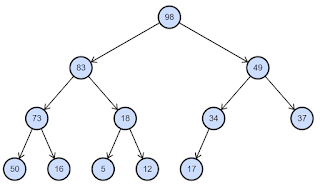# Building Binary Heap In O(n) Worst Time Complexity Explained

There are two ways we can build a binary heap, top-down & bottom-up. We will take top-down approach first. Let’s consider the max heap below.For top-down approach we will start from the root & then move the node towards the bottom till max heap property is satisfied. As you can see from screenshot, heap is a complete binary tree.
We will denote number of nodes at level h as n(h).
When h = 0, n(h) = 2^0 = 1
h = 1, n(h) = 2^1 = 2
h = 2, n(h) = 2^2 = 4
As you can see, a particular level always contains one more node than the total nodes of all previous levels. Let’s consider when height is 2. n(h) is 4 which is greater than (1 + 2) = 3 which is total number of nodes in height 0 & 1.
But because heap is a complete binary tree, last level might not have all the nodes as you can see in the screenshot.
When h = 3, n(h) = 5
But what about total number of leaf nodes? At h=3, we have 5 leaf nodes & h=2 we have 1 leaf node.
So total leaf node is 6. And total non-leaf nodes is also 6. If last level contains all possible nodes, then more than half of total nodes are leaf nodes. Otherwise, we will have at least half nodes as leaf nodes in a complete binary tree. This is an important thing to understand.
Now let’s go back to our top down approach. We start from the root each time. And then move nodes towards leaf level till it satisfies heap property. So we will have to move at least half of the nodes to leaf level from root level which is basically height of the tree h & that is log(n). So this scenario will take (n / 2) * log(n) time. So time complexity will become O(n log(n)).
We will take a look at bottom-up approach now. For bottom-up, we will start at the leaf level. At leaf level, we don’t need to do any shift as any leaf node would satisfy heap property. We move one level up (h-1) & at that level we need to shift at most one level down. At (h-2) level we need to shift at most 2 level.
So we can write something similar as below:
(0 * n/2) + (1 * n/4) + (2 * n/8) + (3 * n/16) + … + (h * 1)
= (1 * n/4) + (2 * n/8) +  (3 * n/16) + … + (h * 1)
h
= ∑ kn/(2^(k+1))
k=1
We can move n out
h
n * ∑ k/(2^(k+1))
k=1
k/(2^(k+1)) can be simplified as k/(2^k * 2). 2 can be omitted as we don’t need to consider constant value for time complexity.
h
∑ k/(2^k)) we need to check what happens when k value approaches to infinity.
k=1
1/ 2^1 + 2/2^2 + 3/2^3 + 4/2^4 + 5/^2^5 + 6/2^6 + ….
=1/2 + 1/2 + 3/8 + 1/4 + 5/32 + 3/32 + ….
As you can see the value will converge towards 2.
So worst time complexity will become O(n * 2) which is basically O(n).